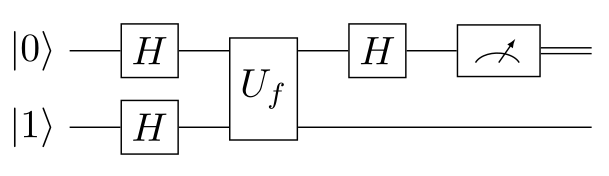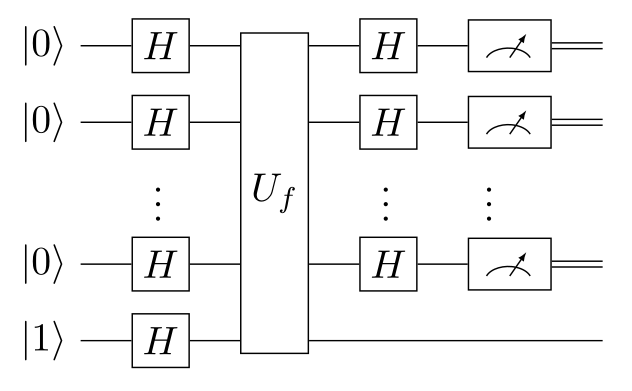Categories: Algorithms, Quantum information.

Deutsch-Jozsa algorithm

The Deutsch algorithm and its extension, the Deutsch-Jozsa algorithm, were first to prove that quantum computers can solve certain problems more efficiently than any classical system.

Given an unknown “black box” binary function $$f(x)$$ of one or more bits $$x$$, the goal is determine whether $$f$$ is constant (i.e. $$f(x)$$ is the same for all $$x$$) or balanced (i.e. exactly 50% of all $$x$$-values yield $$f(x) = 0$$, and the other 50% yield $$f(x) = 1$$). We can query $$f$$ as many times as we want with inputs of our choice, but we want to solve the problem using as few queries as possible.

The problem is extremely artificial and of no practical use, but quantum computers can solve it with a single query, while classical computers need up to $$2^{N - 1} + 1$$ queries for an $$N$$-bit $$x$$.

Deutsch algorithm

The Deutsch algorithm handles the simplest case, where $$x$$ is only a single bit. Only four $$f$$ exist:

• Constant: $$(f(0) = f(1) = 0)$$ or $$(f(0) = f(1) = 1)$$.
• Balanced: $$(f(0) = 0, f(1) = 1)$$, or $$(f(0) = 1, f(1) = 0)$$.

In other words, we only need to determine if $$f(0) = f(1)$$ or $$f(0) \neq f(1)$$. To do this, we use the following quantum circuit, where $$U_f$$ is the oracle we query:Due to unitarity constraints, the action of $$U_f$$ is defined to be as follows, with $$\oplus$$ meaning XOR:

\begin{aligned} \ket{x} \ket{y} \quad \to \boxed{U_f} \to \quad \ket{x} \ket{y \oplus f(x)} \end{aligned}

Starting on the left from two qubits $$\ket{0}$$ and $$\ket{1}$$, we apply the Hadamard gate $$H$$ to both:

\begin{aligned} \ket{0} \ket{1} \quad \to \boxed{H^{\otimes 2}} \to \quad \ket{+} \ket{-} = \frac{1}{2} \Big( \ket{0} + \ket{1} \Big) \Big( \ket{0} - \ket{1} \Big) \end{aligned}

Feeding this result into the oracle $$U_f$$ then leads us to:

\begin{aligned} \to \boxed{U_f} \to \quad \frac{1}{2} \ket{0} \Big( \ket{0 \oplus f(0)} - \ket{1 \oplus f(0)} \Big) + \frac{1}{2} \ket{1} \Big( \ket{0 \oplus f(1)} - \ket{1 \oplus f(1)} \Big) \end{aligned}

The parenthesized superpositions can be reduced. Assuming that $$f(b) = 0$$, we notice:

\begin{aligned} \ket{0 \oplus f(b)} - \ket{1 \oplus f(b)} = \ket{0 \oplus 0} - \ket{1 \oplus 0} = \ket{0} - \ket{1} \end{aligned}

On the other hand, if we assume that $$f(b) = 1$$, we get the opposite result:

\begin{aligned} \ket{0 \oplus f(b)} - \ket{1 \oplus f(b)} = \ket{0 \oplus 1} - \ket{1 \oplus 1} = - \big(\ket{0} - \ket{1}\big) \end{aligned}

We can thus combine both cases, $$f(b) = 0$$ or $$f(b) = 1$$, into the following single expression:

\begin{aligned} \ket{0 \oplus f(b)} - \ket{1 \oplus f(b)} = (-1)^{f(b)} \big(\ket{0} - \ket{1}\big) \end{aligned}

Using this, we rewrite the intermediate state of the quantum circuit like so:

\begin{aligned} \ket{0} \ket{1} \quad \to \boxed{H^{\otimes 2}} \to \boxed{U_f} \to \quad \frac{1}{2} \Big( (-1)^{f(0)} \ket{0} + (-1)^{f(1)} \ket{1} \Big) \Big( \ket{0} - \ket{1} \Big) \end{aligned}

The second qubit in state $$\ket{-}$$ is garbage; it is no longer of interest. The first qubit is given by:

\begin{aligned} \frac{1}{\sqrt{2}} \Big( (-1)^{f(0)} \ket{0} + (-1)^{f(1)} \ket{1} \Big) = \frac{(-1)^{f(0)}}{\sqrt{2}} \Big( \ket{0} + (-1)^{f(0) \oplus f(1)} \ket{1} \Big) \end{aligned}

If $$f$$ is constant, then $$f(0) \oplus f(1) = 0$$, meaning this state is $$(-1)^{f(0)} \ket{+}$$. On the other hand, if $$f$$ is balanced, then $$f(0) \oplus f(1) = 1$$, meaning this state is $$(-1)^{f(0)} \ket{-}$$. Taking the Hadamard transform of this qubit therefore yields:

\begin{aligned} \to \boxed{H} \to \quad (-1)^{f(0)} \ket{f(0) \oplus f(1)} \end{aligned}

Depending on whether $$f$$ is constant or balanced, the mearurement outcome of this state will be $$\ket{0}$$ or $$\ket{1}$$ with 100% probability. We have solved the problem!

Note that we only consulted the oracle (i.e. applied $$U_f$$) once. A classical computer would need to query it twice, once with input $$x = 0$$, and again with $$x = 1$$.

Full Deutsch-Jozsa algorithm

The Deutsch-Jozsa algorithm generalizes the above to $$N$$-bit inputs $$x$$. We are promised that $$f(x)$$ is either constant or balanced; other possibilities are assumed to be impossible. This algorithm is then implemented by the following quantum circuit:There are $$N$$ qubits in initial state $$\ket{0}$$, and one in $$\ket{1}$$. For clarity, the oracle $$U_f$$ works like so:

\begin{aligned} \ket{x_1} \ket{x_2} \cdots \ket{x_N} \ket{y} \quad \to \boxed{U_f} \to \quad \ket{x_1} \cdots \ket{x_N} \ket{y \oplus f(x_1, ..., x_N)} \end{aligned}

Applying the $$N + 1$$ Hadamard gates to the initial state yields the following superposition:

\begin{aligned} \ket{0}^{\otimes N} \ket{1} \quad \to \boxed{H^{\otimes N + 1}} \to \quad \ket{+}^{\otimes N} \ket{-} = \frac{1}{\sqrt{2^N}} \sum_{x = 0}^{2^N - 1} \ket{x} \ket{-} \end{aligned}

Where $$\ket{x} = \ket{x_1} \cdots \ket{x_N}$$ denotes a classical binary state. For example, if $$x = 5 = 2^0 + 2^2$$ in the summation, then $$\ket{x} = \ket{1} \ket{0} \ket{1} \ket{0}^{\otimes N-3}$$ (from least to most significant).

We give this state to the oracle, and, by the same logic as for the Deutsch algorithm, get back:

\begin{aligned} \to \boxed{U_f} \to \quad \frac{1}{\sqrt{2^N}} \sum_{x = 0}^{2^N - 1} (-1)^{f(x)} \ket{x} \ket{-} \end{aligned}

The last qubit $$\ket{-}$$ is garbage. Next, applying the Hadamard transform to the other $$N$$ gives:

\begin{aligned} \to \boxed{H^{\otimes N}} \to \quad \frac{1}{\sqrt{2^N}} \sum_{x = 0}^{2^N - 1} (-1)^{f(x)} \bigg( \frac{1}{\sqrt{2^N}} \sum_{y = 0}^{2^N - 1} (-1)^{x \cdot y} \ket{y} \bigg) \end{aligned}

Where $$x \cdot y$$ is the bitwise dot product of the binary representations of $$x$$ and $$y$$, so, for example, if $$N = 2$$, then $$x \cdot y = x_1 y_1 + x_2 y_2$$. Note that the above expression has not been reduced at all; it follows from the definition of the Hadamard transform. We can rewrite it like so:

\begin{aligned} \frac{1}{2^N} \sum_{x = 0}^{2^N - 1} \sum_{y = 0}^{2^N - 1} (-1)^{f(x) + x \cdot y} \ket{y} = \sum_{y = 0}^{2^N - 1} \bigg( \frac{1}{2^N} \sum_{x = 0}^{2^N - 1} (-1)^{f(x) + x \cdot y} \bigg) \ket{y} = \sum_{y = 0}^{2^N - 1} c_y \ket{y} \end{aligned}

The parenthesized expression can be interpreted as the coefficients of a superposition of several $$y$$-values. Therefore, the probability that a measurement yields $$y = 0$$, i.e. $$\ket{y} = \ket{0}^{\otimes N}$$, is:

\begin{aligned} |c_0|^2 = \bigg| \frac{1}{2^N} \sum_{x = 0}^{2^N - 1} (-1)^{f(x)} \bigg|^2 \end{aligned}

The summation always contains an even number of terms, for all values of $$N$$. Consequently, if $$f$$ is constant, then $$|c_0|^2 = |\!\pm\! 2^N / 2^N|^2 = 1$$. Otherwise, if $$f$$ is balanced, all the terms cancel out, so we are left with $$|c_0|^2 = 0$$. In other words, we reach the same result as the Deutsch algorithm: we only need to measure the $$N$$ qubits once; $$f$$ is constant if and only if all are zero.

The Deutsch-Jozsa algorithm needs only one oracle query to give an error-free result, whereas a classical computer needs $$2^{N-1} + 1$$ queries in the worst case; a revolutionary discovery.

1. J.S. Neergaard-Nielsen, Quantum information: lectures notes, 2021, unpublished.
2. S. Aaronson, Introduction to quantum information science: lecture notes, 2018, unpublished.

© Marcus R.A. Newman, a.k.a. "Prefetch". Available under CC BY-SA 4.0.